Mathematical Theory of Space-Time | OMICS International
Journal of Physical Mathematics

# Mathematical Theory of Space-Time

Dr. Andreas Bacher*

Faculty of Mathematics and Natural Sciences, University of Cologne, Albertus-Magnus-Platz, Germany

*Corresponding Author:
Dr. Andreas Bacher
Faculty of Mathematics and Natural Sciences
University of Cologne,Albertus-Magnus-Platz, Germany
Tel: 21561646
E-mail: [email protected]

Received Date: December 16, 2016; Accepted Date: Janaury 20, 2017; Published Date: Janaury 25, 2017

Citation: Bacher A (2017) Mathematical Theory of Space-Time. J Phys Math 8: 210. doi: 10.4172/2090-0902.1000210

Copyright: © 2017 Bacher A. This is an open-access article distributed under the terms of the Creative Commons Attribution License, which permits unrestricted use, distribution, and reproduction in any medium, provided the original author and source are credited.

Visit for more related articles at Journal of Physical Mathematics

#### Abstract

We hypothesize that the Universe contains observable subluminal matter (tardyons and locatily) and unobservable superluminal matter (tachyons and nonlocality). By using space-time ring we establish mathematical theory of space-time with subluminal and superluminal coexistence. The rotating motions of tardyons and tachyons produce centrifugal and centripetal (gravity) forces, respectively. This paper deduces a new gravitational formula and Newtonian gravitational formula from the tardyonic and tachyonic coexistence principle. Through the morphism we first show that tardyons and tachyons are interchangeable, but that tachyons are unobservable . We then convert the tachyonic mass into tardyonic mass We obtain gravitational coefficient η = 6.9 ×10-10.Using it we establish the expansion theory of the universe and suggest the new universe model

#### Keywords

New gravitational formula; Expansion theory of universe; New universe model

#### Introduction

Through the morphism we first show that tardyons and tachyons are interchangeable, but that tachyons are unobservable. We then convert the tachyonic mass into tardyonic mass we obtain gravitational coefficient η=6.9 × 10-10. Using it we establish the expansion theory of the universe and suggest the new universe model.

#### Mathematical Theory of Space-Time

In the Universe there are two matters: (1) observable subluminal matter called tardyon (locality) and (2) unobservable superluminal matter called tachyon (non-locality) which coexist in motion. Tachyon can be converted into tardyon, and vice versa. Tardyonic rotating motion produces the centrifugal force, but tachyonic rotating motion produces the centripetal force, that is gravity. Using tardyonic and tachyonic coexistence principle we deduce the new gravitational formula. For establishing the mathematical theory of space-time we first define space-time ring [1-3].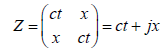(1)

where x and t are the tardyonic space and time coordinates, c is light velocity in vacuum,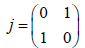(1) can be written as Euler form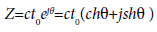(2)

From (1) and (2) we have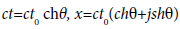(3)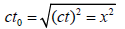(4)

From (3) we have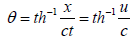(5)

where c ≥ u is the tardyonic velocity.

Using the morphism j: z → jz, we have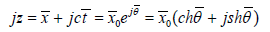(6)

Where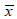and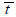are the tachyonic space and time coordinates,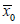is tachyonic invariance,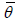tachyonic hyperbolical angle.

From (6) we have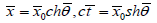(7)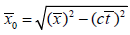(8)

From (7) we have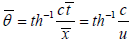(9)

where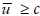is the tachyonic velocity

Figure 1 shows the formulas (1)-(9). j: z → jz is that tardyon can be converted into tachyon, but j: jz → z is that tachyon can be converted into tardyon. u=0→u=c is the positive acceleration, but u=∞ →u=c is the negative acceleration, which coexist. At the ct-axis u=0 and x=0 we define the tardyonic test time t. At the x − axis we define the tachyonic rest space as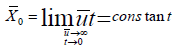(10)

Since at rest the tachyonic rest time t=0 and u=∞, we prove that tachyon is unobservable. Figure 1 and (10) are mathematical theory of space-time, which are the foundations of physics andcosmology. Using it we prove that dark matter, dark energy, gravitational waves, black holes, quantum entanglement and quantum computers do not exist. From Figure 1 we deduce new gravitational formula.

#### New Gravitational Formula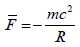Assume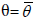, from (5) and (9) we get the tardyonic and tachyonic coexistence principle [1-6]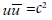(11)

Using the geometrical method we deduce the new gravitational formula.

Figure 2 shows that the rotation ω of body A emits tachyon mass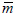, which forms the tachyon and gravitation field and gives the body B revolutions u and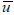.

From Figure 2 it follows

Figure 2: On body B the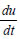and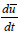coexistence.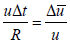(12)

From (12) it follows the tardyon centripetal acceleration on the body B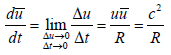(13)

From Figure 2. it follows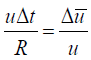(14)

From (14) and (11) it follows the tachyon centrifugal acceleration on the body B [4,7]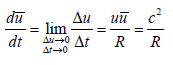(15)

From (13) it follows the tardyon centrifugal force on body B [2-4],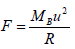(16)

where MB is body B mass.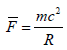(17)

From (15) it follows the tachyon centripetal force on body B, that is gravity [8-10],

Where m is the gravitation mass converted into by tachyon mass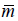, which is unobservable, but m is observable.

(17) Is the new gravitational formula. This simple thought made a deep impression on me. It impelled me to establish the new gravitational theory explained in Figure 3.

Figure 3: On body B the F and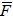coexistence.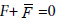(18)

From (16), (17) and (18) it follows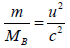(19)

Body B increases mass m and centrifugal force is greater than gravitation force, then body B expands outward. Dark matter which causes cosmic attraction is wrong. From (17) it follows Newtonian gravitation formula. The m is proportional to body A mass MA, in (19) m is proportional to MB, is inversely proportional to the distance R between body A and body B. It follows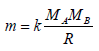(20)

where k is constant

Substituting (20) into (17) it follows the Newtonian gravitational formula [10-12]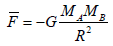(21)

where G=kc2=6.673 × 10-8 cm3/ g.sec2 is gravitation constant.

Now we study the freely falling body. Tachyonic mass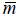can be converted into tardyonic mass m, which acts on the freely falling body and produces the gravitational force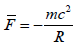(22)

where R is the Earth radius.

We have the equation of motion(23)

where g is gravitational acceleration, M is mass of freely falling body.

From (23) it follows the gravitational coefficient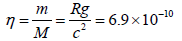(24)

Eötvös experiment η∼5.10-9 and Dicke experiment η∼10-11. Since the gravitational mass m can be transformed into the rest mass in freely falling body, we prove that the freely falling bodies fall with the same acceleration.

#### The Expansion Theory of the Universe

Using new gravitational formula we study the expansion theory of the Universe . Figure 4 shows an expansion model of the Universe. The rotation ω1 of body A emits tachyonic flow, which forms the tachyonic field. Tachyonic mass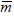acts on body B, which produces its rotation ω2, revolution u and gravitational force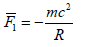(25)

where R denotes the distance between body A and body B, m is gravitational mass converted into by tachyonic mass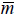which is unobservable but m is observable. The rotation of the body B around body A produces the centrifugal force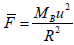(26)

where MB is the inertial mass of body B, u is the orbital velocity of body B .

At the O2 point we assume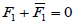(27)

From (27) it follows that the coexistence of the gravitational force and centrifugal force.

From (25)-(27) it follows the gravitational coefficient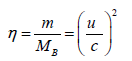(28)

At the O3 point the tachyonic mass m can be converted into the rest mass m in body B, it follows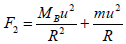(29)

Since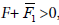centrifugal force F2 is greater than gravitational force F1, then the body B expands outwards and its mass increases. This is an expansion mechanism of the Universe. From (26,27,29) we have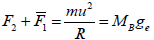(30)

From (30) we obtain the expansion acceleration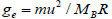(31)

Substituting (28) in (31) we obtain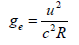(32)

If body A is the Earth, then body B is the Moon; if body A is the Sun, then body B is the Earth; …. It can explain our accelerating universe. In the universe there are no dark matter and no dark energy. This simple thought made a deep impression on me. It impelled me to establish an expansion theory of the universe. Dark energy responsible cosmic repulsion is wrong. If the body A is the Sun and body B is the planet. We calculate the gravitational coefficients η as shown in Table 1. The gravitational field of the solar system is the origin of the planet mass. From it the planet acquire mass.

Planet u (km/sec) η(10−10 )
Venus 35.03 136.5
Earth 29.79 98.7
Mars 24.13 64.8
Jupiter 13.06 19
Saturn 9.64 10.3
Uranus 6.81 5.2
Neptune 5.43 3.3
Pluto 4.74 2.5

Table 1: Values of the gravitational coefficients η.

#### The New Universe Model

From the tachyonic theory we suggest the new universe model. The universe has no beginning and no end. The universe is infinite, but it has a center consisting of the tachyonic matter which is strong gravitational field (SGF), which governs motion of the whole universe. Therefore the whole universe is stable and harmonious. … .In the sun there is a center consisting of the tachyonic matter with SGF, which governs motion of the solar system. It is stable and harmonious. In the earth there is a center consisting of the tachyonic matter with SGF, which governs motion of the earth and the moon. It is stable and harmonious. In the moon there is a center consisting of the tachyonic matter with SGF, which governs motion of the moon. It is stable and harmonious. In atomic nucleus there is a center consisting of the tachyonic matter with SGF, which governs motion of the nucleus. Therefore atomic nuclei are stable and harmonious. The tachyonic theory governs the amazing harmony of the whole universe from the smallest to the largest scales. New gravitational formula changes all that. In the Universe there are no dark matter, no dark energy and no gravitational waves. Multiverse, inflation and primordial gravitational waves do not exist .

#### Conclusion

We deduce tardyonic and tachyonic coexistence principle. Using it we deduce the centrifugal formula and new gravitational formula. We establish the expansion theory of the universe without dark energy and suggest the new universe model which is amazing harmony. The new gravitational formula is foundations of particle physics and cosmology. We prove that in the universe no dark matter, no dark energy, no gravitational waves and no quantum gravity. . Where did we come from? Where are we going? What makes up the universe? These questions have occupied mankind for thousands of years. Over the course of history, our view of the world has been changed. Theologians and philosophers, physicists and astronomers have given us very different answers. Where did we come from? We answer this questions this way m → m, Tachyons → tardyons, that is tachyons can be converted into the electrons and positrons which are the basic building-blocks of the elementary particles. The tachyons are the origin of mass. Where are we going? We answer this question this way m → m, that is the tardyons produce tachyons. The tardyons and tachyons make up the Universe.

#### References

Select your language of interest to view the total content in your interested language

### Article Usage

• Total views: 7194
• [From(publication date):
March-2017 - Jan 24, 2020]
• Breakdown by view type
• HTML page views : 6781Can't read the image? click here to refresh